# A easy method to do a batch decryption of APP strings

Published:
This guild introduces an easy method to decrypt the kind of strings and write the decrypted string back to the decompiling code.

## Introduction

Important strings in some APKs, especially Android malware, are encrypted to increases the difficulty of reverse engineering. The number of such strings is often large and it is difficult to decrypt them one by one. This guild introduces an easy method to decrypt the kind of strings and write the decrypted string back to the decompiling code.

Here, I will finish this kind of work with GDA (GDA is a Dalvik bytecode decompiler written entirely in c++, no relay on java, supports decompilation of APK, DEX, ODEX, OAT, JAR, AAR, and CLASS  files), The case of APKs is from malware (Samples are not provided to prevent the spread of risks). The malware information is as follows:

```[File Base Info]
File Name:      C:\Users\**\fish.apk
Package Name:   fdsvcc.bcxa.bvdwq
Main Activity:  fdsvcc.bcxa.bvdwq.MainActivity
File Size:      2631444 bytes
Packed:         Not Packed
Min SDK:        15 Target
SDK:            28```

Python decrypting script code: script one, script two

Java decrypting script code(in zip): script one and two

## Decryption with Python

1. Through GDA > AllString, we can see that a large number of strings of the app are encrypted. As shown in the figure: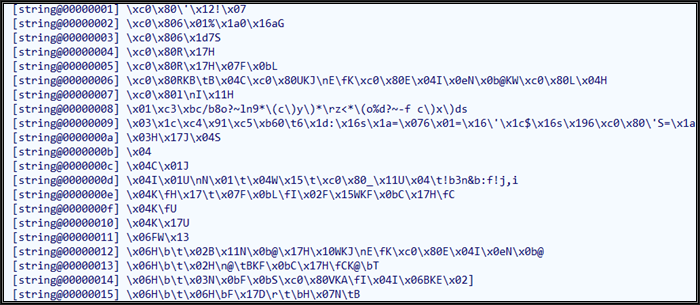There are thousands of encrypted strings, so we need to decrypt them automatically with a decryption script. Moving the cursor into [] and pressing x (string cross-reference), we can clearly see the decryption method. One of the decryption in the red rectangle box as follows: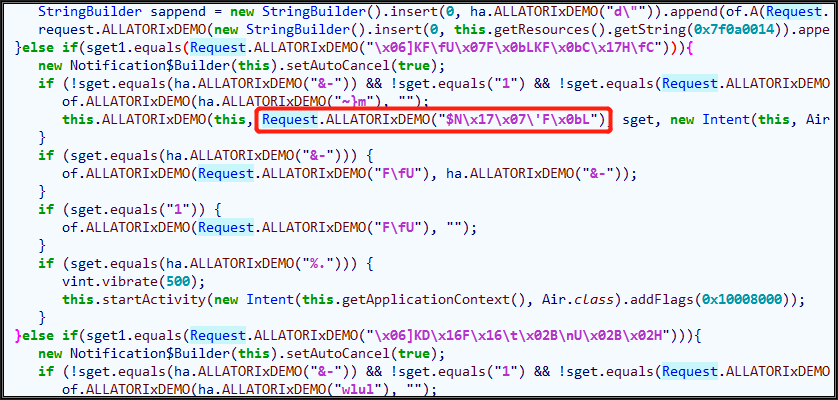We will use this function as an example to decrypt all strings associated with it. Double click the function Request.ALLATORIxDEMO to decompile the function code: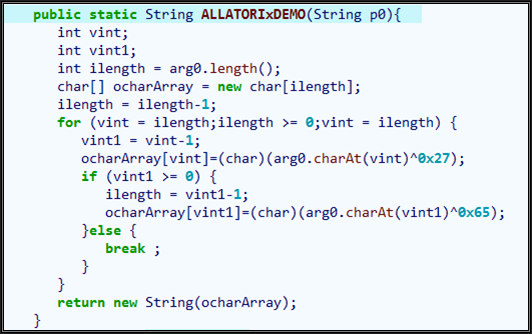As the above figure shows, the decryption method iterates from the end of the string, XOR calculation is performed with 0x27 and 0x65 respectively, so the implementation is simple.

2. Our goal is to decrypt all the strings associated with the decryption method. Therefore, We can do it through the following steps:

• First, use Python to re-implement Request.ALLATORIxDEMO(String p0).
• Next, you need to locate the decryption method in the APP and find all the callers.
• Last, extract the encrypted string from the caller, and decrypt it and write back to the APP(do not modify the original APK file)

So, let's begin.

1) Implementation of decryption function Python

It is also very simple to implement the decryption algorithm in Python, as follows:

```def decodeString(gda,idx):
rawstr=gda.GetStringById(idx)
if rawstr==None:
return ''
stri=rawstr
ret=list(stri)
i=len(stri)-1
xx=''
while i>= 0:
ret[i]=chr(ord(stri[i])^39) #0x27
if i <= 0:
break
i=i-1
ret[i]=chr(ord(stri[i])^101) #0x65
i=i-1
xx = ''.join(ret)
return xx```

2) Locate the decryption method

First, we need to find the decryption method and its caller. In the decompiled Request.ALLATORIxDEMO method, press F5 to compile the Smali code, as shown in the following figure: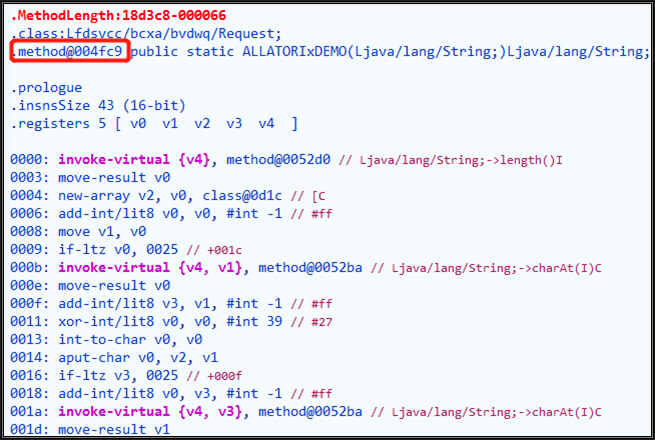The index of the method (the index is the index of the method in the DEX file) is 004fc9, and then we can locate the function through the following code.

```def GDA_MAIN(gda_obj):
gda=gda_obj             #GDA object
Dex0=gda.DexList     #The first DEX of apk
midx=0x4fc9             #method idx
method=Dex0.MethodTable[str(midx)] #Find the method quickly through methodTable.```

Here, the method object (class methodinfo) is the decryption function we are looking for. callorIdxList of method object (see GdaImport.py File) properties are all callers of the method. All callers can be accessed by traversing the list callorIdxList.

3) Locate string and decrypt

After finding the caller, we also need to find the position of the decryption method in the caller function to further locate the encrypted strings. Let's go back to GDA and look at the context in which the caller calls the decryption method.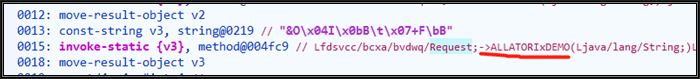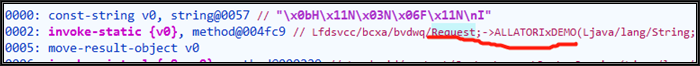We can see that encrypted strings appear above Request.ALLATORIxDEMO. Therefore, we disassemble the caller (through python API GetSmaliCodeById) to get smali, and then reverse locate the strings from the line where the decryption method is called, and extract the index of the string for decryption. The python code is as follows (the dictionaries callorTable={} and strIdxTable={} are added to the original python code To prevent repeated process).

```clist=method.callorIdxList
destr=''
for idx in clist:
smalicode=gda.GetSmaliCodeById(idx)
splitstr=smalicode.split('\r\n')
i=0
for sstr in splitstr:
if '@004fc9' in sstr:
line=splitstr[i-1]
if 'string@' in line:
pos=line.find('ing@')+4
strIdx=line[pos:pos+4]
dstr=decodeString(gda,int(strIdx,16))
gda.SetStringById(int(strIdx,16),dstr)
destr+="[string@"
destr+=strIdx
destr+="] "
destr+=dstr
destr+='\n'
i=i+1   ```

Code annotation: first traverse the callorIdxList, which stores the indexes of the caller methods. We can use the Python API GetSmaliCodeById disassemble the method, then split the smali code into lines, and locate the row of the decryption method through'@004fc9', then reverse look for the strings that need to be decrypted, extract the indexes of the strings, finally call the decryption function re-implemented by python to decrypt them and write back through python API SetStringById. In order to cross-reference for the decrypted strings, I added a string interaction flag[string@xxxx]. running the python script and see the results: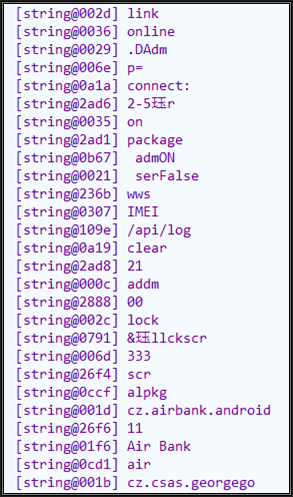And the strings in the decompiling code is also clear.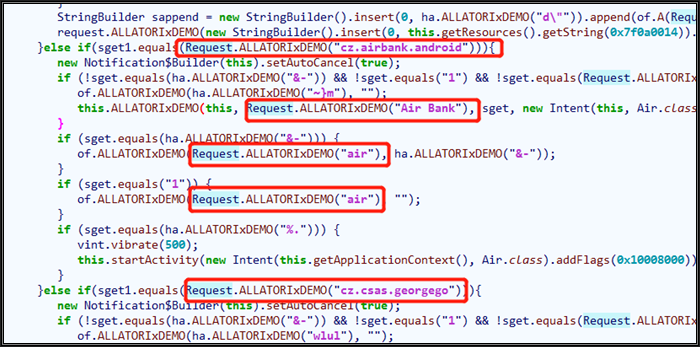The above method is simple and easy to understand. There is a faster method to do the same thing. We may not use disassembling API(GetSmaliCodeById) but the bytecode data API(DumpHexData) to locate the encrypted strings. See the code for details. In the code, API DumpHexData is used to extract the bytecode of the caller method (represented in the form of a hexadecimal string), and then the indexes of the strings to be decrypted is located through the bytecode feature.

How to locate features? Use the above method to locate one of the caller functions, and then compile it into Smali. Right-click > show bytecode to analyze the location characteristics of decrypted strings: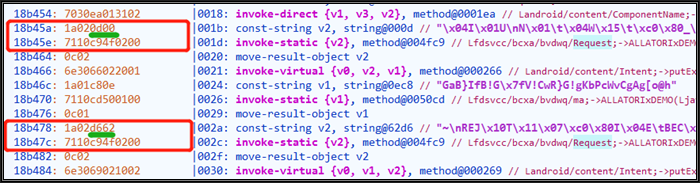First, find "c94f" (0x4fc9), then forward to the encrypted string index.

## Decryption using java

GDA provides the same interface for java, which will not be discussed in detail. we just need to pay attention to the following two points.

1. When using java to decrypt strings, if the decryption method only uses the java foundation library, the decompiled code can be directly used to decrypt.
2. Since Java virtual machine cannot be dynamically released and overloaded after it is loaded into a process, java code with the same class name cannot be run repeatedly in a GDA process.

0
122 Views